# Examples for 7th grade (seventh)

1. Lcm of three numbersWhat is the Lcm of 120 15 and 5
2. One deciWine 0,375 l costs 2040 CZK. How much does 1 dl cost?
3. Two boats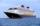The sightseeing boat sail at 25 km / h. The cruise take 6 hours. How long the another boat sails at 60 km/h?
4. Pond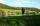A pond with two open floodgates will emptied in 20 days. A larger floodgate will emptied whole pond in 30 days. For how long would he be emptied with a smaller floodgate.A 2-meter rod casts a shadow 3.2 m long. How high is a tree with a shadow of 14.4 m ?
6. PumpingThe tank is filled with a larger pump in 12 hours and with scond pump in 15 hours. For how long does the tank fill when both pumps are switched on at the same time?
7. TrenchThe trench is a four-sided prism. The cross section has a trapezoidal shape with basements of 4m and 6m, the length of the trench is 30m. What is the depth of the trench if we dig 60,000 l of soil.
8. Pins 2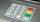how many different possible 4 digits pins can be found on the 10-digit keypad?
9. A mapA map of size 60cm by 25cm is reduced in the ratio of 3:5, twice. What are the new dimensions?
10. CuboidCuboid has a surface of 516 cm2. Side a = 6 cm and b = 12 cm. How long is the side c =?
11. Surface of cuboidFind the surface of the cuboid if its volume is 52.8 cm3 and the length of its two edges is 2 cm and 6 cm.
12. Type of triangle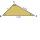How do I find the triangle type if the angle ratio is 2:3:7 ?
13. Volume of cubeSolve the volume of a cube with width 26cm .
14. Inter city busThe inter city bus leaves Suva at 10.00am and reaches Nadi at 1.00pm covering a distance of 219km. How long did it take to reach the bus Nadi?
15. Trapezoidal prismCalculate the surface of the quadrilateral prism ABCDA'B'C'D 'with the trapezoidal base ABCD. The height of the prism is 12 cm; ABCD trapezoidal data: AB base length is 8 cm, CD base length is 3 cm, BC arm length is 4 cm, and AC diagonal length is 7 cm.
16. Pills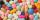If it takes 20 minutes to run a batch of 100 pills how many minutes would it take to run a batch of 50 pills
17. Tailor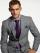From the rest of the cloth tailor could cut off either 3 m in men's suits without vest or 3.6 m with vest. What shortest possible length could the rest of the cloth have? How many suits a) without a vest b) with vest could make the tailor from the r
18. Guess a fractionTom was asked to guess a fraction. The sum of 1/2 the numerator and 1/3 of its denominator is 30. If Tom subtracts 36 from its denominator, the fraction becomes 1/3. What is the fraction that Tom was asked to guess? (Leave your answer in simplest form)
19. Roses 2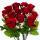Aunt Rose went to the flower shop to buy a bouquet with three roses. The flower shop had white, yellow and red roses. How many different flowers bouquet can a flower make for Aunt Rose create? Write all the bouquet options.
20. Triangular prismCalculate the volume and surface of the triangular prism ABCDEF with base of a isosceles triangle. Base's height is 16 cm, leg 10 cm, base height vc = 6 cm. The prism height is 9 cm.

Do you have an interesting mathematical word problem that you can't solve it? Submit math problem, and we can try to solve it.

We will send a solution to your e-mail address. Solved examples are also published here. Please enter the e-mail correctly and check whether you don't have a full mailbox.

Please do not submit problems from current active competitions such as Mathematical Olympiad, correspondence seminars etc...